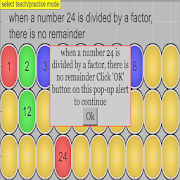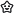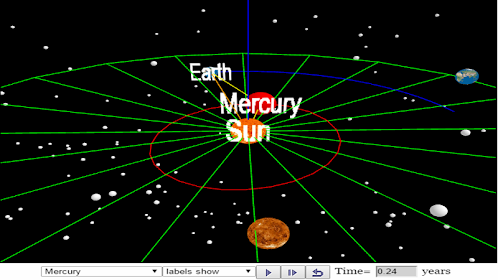# Even Odd Multiples Factors SimulatorEveryoneFamily Friendly
An open source physics at Singapore simulation based on codes written by Loo Kang WEE
more resources can be found here
http://iwant2study.org/ospsg/index.php/interactive-resources/mathematics

Topics
Primary 2
1 WHOLE NUMBERS
Numbers up to 10 000
Include:
• number notation and place values (thousands, hundreds, tens, ones),
• reading and writing numbers in numerals and in words,
• comparing and ordering numbers,
• odd and even numbers,
• number patterns

Primary 4
1 Whole Numbers
Factors and multiples
Include:
• determining if a 1-digit number is a factor of a given number,
• listing all factors of a given number up to 100,
• finding the common factors of two given numbers,
• recognising the relationship between factor and multiple,
• determining if a number is a multiple of a given 1-digit number,
• listing the first 12 multiples of a given 1-digit number,
• finding the common multiples of two given 1-digit numbers.
Exclude 'highest common factor' (H.C.F.) and 'lowest common multiple’ (L.C.M.).

Primary 5 Foundation Mathematics (Calculator is allowed unless otherwise stated)
1 Whole Numbers
Factors and multiples
Include:
• determining if a 1-digit number is a factor of a given number,
• listing all factors of a given number up to 100,
• finding the common factors of two given numbers,
• recognising the relationship between factor and multiple,
• determining if a number is a multiple of a given 1-digit number,
• listing the first 12 multiples of a given 1-digit number,
• finding the common multiples of two given numbers up to 12.
Exclude 'highest common factor' (H.C.F.) and 'lowest common multiple’ (L.C.M.).

reference:
https://www.moe.gov.sg/docs/default-source/document/education/syllabuses/sciences/files/2007-mathematics-(primary)-syllabus.pdf

Interesting Fact
This simulation written by ordinary educator teacher, i am not a professional programmer or vendor.

Project
SSTRF_2017_ETD_3 Explore-Useful Learning Math Apps, please contact weelookang@gmail.com to participate in testing the apps for Primary Math Singapore.

Acknowledgement
My sincere gratitude for the tireless contributions of Francisco Esquembre, Fu-Kwun Hwang, Wolfgang Christian, Félix Jesús Garcia Clemente, Anne Cox, Andrew Duffy, Todd Timberlake and many more in the Open Source Physics community.
Collapse

Review Policy

## What's New

updated to ionic 4
Collapse

Updated
July 8, 2019
Size
9.2M
Installs
500+
Current Version
0.0.15
Requires Android
4.4 and up
Content Rating
Everyone
Permissions
Offered By
Open Source Physics Singapore
Developer